# em.glm vignette

## Introduction

‘em.glm’ is a package that fits Expectation Maximization glm regression via an iterative reweighted least squares approach. The underlying algorithm is used to find the (local) maximum likelihood parameters of a system with hidden variables. Notably, it allows for the modelling of a mixture model by assuming that each observed data point has a corresponding unobserved feature corresponding to which model holds true.

## Installation

At present the package is available in development state from GitHub:

install.packages("devtools")
devtools::install_github("Stat-Cook/emax.glm")

## Quick Start

The majority of the heavy lifting of the package relies on the ‘em.glm’ function. The function requires a matrix of covariates (x), a target vector (y), a GLM family (defaulting to poisson()) and the number of target classes (K). Generally taking the form:

em.glm(
x, y,
family = poisson(),
K = 2
)

The maximization algorithm used here relies on local gradient descent and hence can struggle with local minima. Generally we would counteract local minima by performing the fit from multiple starting states and comparing the finalized models however, the EM approach can be time consuming to converge. The solution to this we supply here is the ‘small.em’ function which runs several early-stopping trials of the EM algorithm from varying starting states. We can then take the optimal of these as the starting parameter space for the ‘em.glm’ command. The work flow for this warm-up approach is:

warm.up <- small.em(
x, y,
b.init = "random", K = K,
repeats = 20
)

params <- select_best(warm.up)

fit.K2 <-  em.glm(
x, y ,
K = 2, b.init = params
)

## In practice

To help new users - here we demonstrate a basic application of the EM algorithm - for more in-depth uses or for the underlying mathematics the reader is recommended to read the other vignettes.

We have previously simulated a data set (sim.2) consisting of 5 features V1, V2, V3, V4, V5 and a target feature $$y$$. This data set was simulated from 2 competing processes, with an underlying Poisson process. A quick Poisson regression shows the key issue:

x <- sim.2$data[, 1:5] y <- sim.2$data[, 6]

pois.glm <- glm(y ~ . , data = sim.2$data, family = poisson())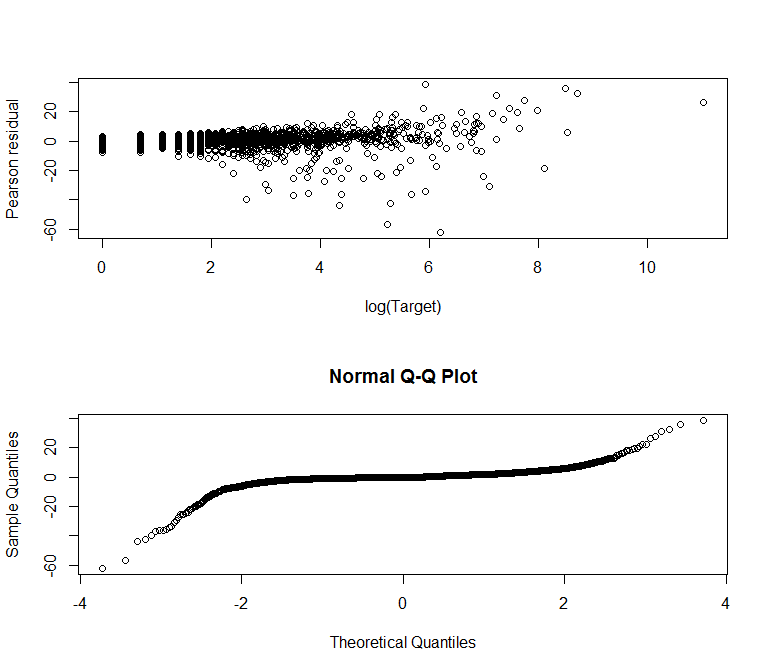Residual diagnostic plots for Poisson fit despite the data having been simulated from a Poisson process - the Pearson residuals show over-dispersion and strong tails at the extremities of the QQ plot. Classically we might say the data is simply over-dispersed and apply a correction, but in fact there may be two competing models. To check, we apply the EM algorithm here (excluding parameter errors): library(emax.glm) df <- sim.2$data

x <- as.matrix(df[, 1:5])
y <- df$y pois.em <- em.glm(x = x, y = y, K = 2, b.init = "random", param_errors = FALSE) dev.residuals <- residuals(pois.em, x = x, y = y, type = "deviance") kable(summary(pois.em)) Parameter Error K1 - X1 -1.5708679 - K1 - X2 -0.1037010 - K1 - X3 -1.7631339 - K1 - X4 -1.4350861 - K1 - X5 -0.0009207 - K2 - X1 -1.3611519 - K2 - X2 0.2717299 - K2 - X3 -1.3721967 - K2 - X4 -0.5871682 - K2 - X5 -1.0550809 - Accessing the model deviance residuals. We can see that the model gives better residuals had we expected a normal distribution: qqnorm(dev.residuals)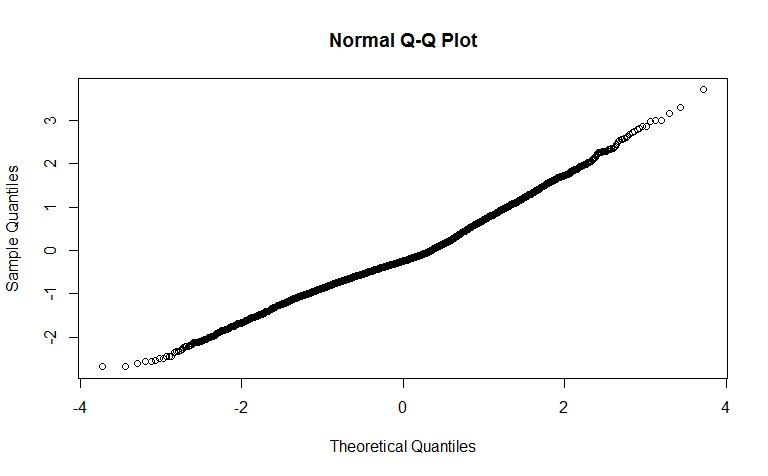Normality of EM-Poisson residuals The r plot’ command has been updated to plot the predicted values of each input variable as a line graph, and here we include the known parameters (from the simulation stage) for comparison: { par(mfrow = c(2,1)) plot(pois.em, known_params = sim.2$p1)
plot(pois.em, known_params = sim.2$p2) }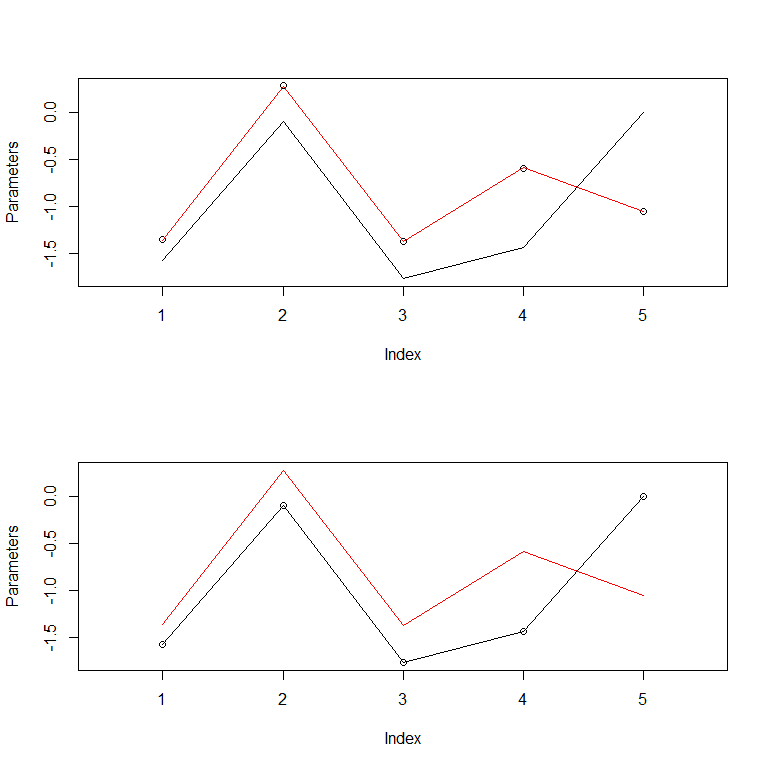Predicted and known parameters Showing we are in good agreement with the underlying model. This can be seen clearly if we call on the model quality metrics such as AIC or BIC: quality <- data.frame( glm = c(AIC(pois.glm), BIC(pois.glm)), em = c(AIC(pois.em), BIC(pois.em)) ) rownames(quality) <- c("AIC", "BIC") kable(quality) glm em AIC 80211.53 13957.06 BIC 80250.64 13983.13 ## Effect of too many classes Clearly the use of the EM approach relies on the analysts choice of how many underlying classes should be included (tuning $$K$$). We have seen what might happen to the model if we fit with too few classes - but what about too many? Here we fit a second simulated data set, {r, eval = FALSE} sim.1 - similar to the previous behavior but with only a single underling Poisson process. We first establish the true model: pois.glm <- glm(y ~ ., data = sim.1$data, family = poisson())
qqnorm(residuals(pois.glm, type = "deviance"))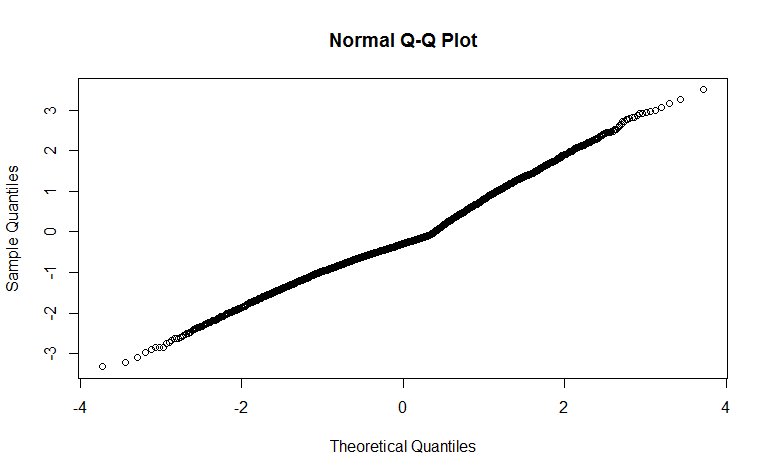and see normal-Pearson residuals as we might expect.

df <- sim.1$data x <- as.matrix(df[,1:5]) y <- df$y

pois.em <- em.glm(
x, y,
family = poisson(),
b.init = "random",
K = 2
)

kable(summary(pois.em))`
Parameter Error
K1 - X1 0.5057757 -
K1 - X2 -0.8021451 -
K1 - X3 -0.1847351 -
K1 - X4 1.5895225 -
K1 - X5 -1.3863162 -
K2 - X1 0.4942698 -
K2 - X2 -0.7759452 -
K2 - X3 -0.1936245 -
K2 - X4 1.6048992 -
K2 - X5 -1.3765934 -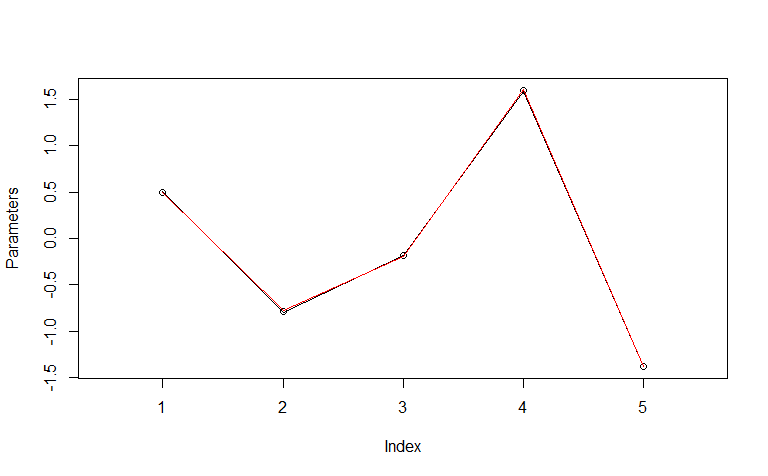Single parameter set EM fit

we see that the model has collapsed both sets of parameters to the the same values. Setting too many classes doesn’t pose a large issue beyond the computational time.